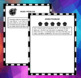# HOW TO MAKE EQUIVALENT FRACTIONSSubject
Resource Type
Product Rating
File Type

Zip

(4 MB|34 pages)
Also included in:
1. This product is composed of three units: How To Represent A Fraction, How To Compare And Order Fractions, and How To Make Equivalent FractionsIn the first unit (How To Represent A Fraction), students will learn how to represent a fraction in a variety of ways. Each lesson contains a MINDS ON ACTIVIT
\$15.00
\$11.10
Save \$3.90
Product Description

This unit includes:

• 5 LESSONS
• 5 EXIT CARDS
• 5 EXTRA PRACTICE WORKSHEETS
• 2 HOW-TO REUSABLE FRAMEWORKS with STEP-BY-STEP instructions

In this unit students will develop an understanding of equivalent fractions using common denominators. Lessons are designed so that students begin working with concrete materials, then move to pictorial drawings to represent their thinking, and finally solve for equivalent fractions using numbers only.​

Each lesson contains a MINDS ON TASK CARD, an EXIT CARD, and an EXTRA PRACTICE WORKSHEET.

Lessons have MODELLED examples and opportunities for SHARED, GUIDED, and INDEPENDENT practice.

LESSON 1

How To Make Equivalent Fractions Using Fraction Strips

LESSON 2

How To Make Equivalent Fractions Using Counters (Arrays/Ratios)

• Includes a HOW TO reusable worksheet with STEP-By-STEP instructions

LESSON 3

How To Make Equivalent Fractions Using Pictorial Drawings

LESSON 4

How To Make Equivalent Fractions Using Numbers Only

• Includes a HOW TO reusable worksheet

LESSON 5

How To Solve Problems Involving Equivalent Fractions

Total Pages
34 pages
Included
Teaching Duration
Other
Report this Resource to TpT
Reported resources will be reviewed by our team. Report this resource to let us know if this resource violates TpT’s content guidelines.